# 8. Scapegoat Trees

In this chapter, we study a binary search tree data structure, the ScapegoatTree. This structure is based on the common wisdom that, when something goes wrong, the first thing people tend to do is find someone to blame (the scapegoat). Once blame is firmly established, we can leave the scapegoat to fix the problem.

A ScapegoatTree keeps itself balanced by partial rebuilding operations. During a partial rebuilding operation, an entire subtree is deconstructed and rebuilt into a perfectly balanced subtree. There are many ways of rebuilding a subtree rooted at nodeinto a perfectly balanced tree. One of the simplest is to traverse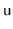's subtree, gathering all its nodes into an array,, and then to recursively build a balanced subtree using. If we let, then the element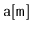becomes the root of the new subtree,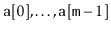get stored recursively in the left subtree andget stored recursively in the right subtree.

```    void rebuild(Node<T> u) {
int ns = size(u);
Node<T> p = u.parent;
Node<T>[] a = (Node<T>[]) Array.newInstance(Node.class, ns);
packIntoArray(u, a, 0);
if (p == nil) {
r = buildBalanced(a, 0, ns);
r.parent = nil;
} else if (p.right == u) {
p.right = buildBalanced(a, 0, ns);
p.right.parent = p;
} else {
p.left = buildBalanced(a, 0, ns);
p.left.parent = p;
}
}
int packIntoArray(Node<T> u, Node<T>[] a, int i) {
if (u == nil) {
return i;
}
i = packIntoArray(u.left, a, i);
a[i++] = u;
return packIntoArray(u.right, a, i);
}
Node<T> buildBalanced(Node<T>[] a, int i, int ns) {
if (ns == 0)
return nil;
int m = ns / 2;
a[i + m].left = buildBalanced(a, i, m);
if (a[i + m].left != nil)
a[i + m].left.parent = a[i + m];
a[i + m].right = buildBalanced(a, i + m + 1, ns - m - 1);
if (a[i + m].right != nil)
a[i + m].right.parent = a[i + m];
return a[i + m];
}
```
A call to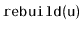takestime. The resulting subtree has minimum height; there is no tree of smaller height that has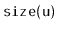nodes.

Subsections
opendatastructures.org